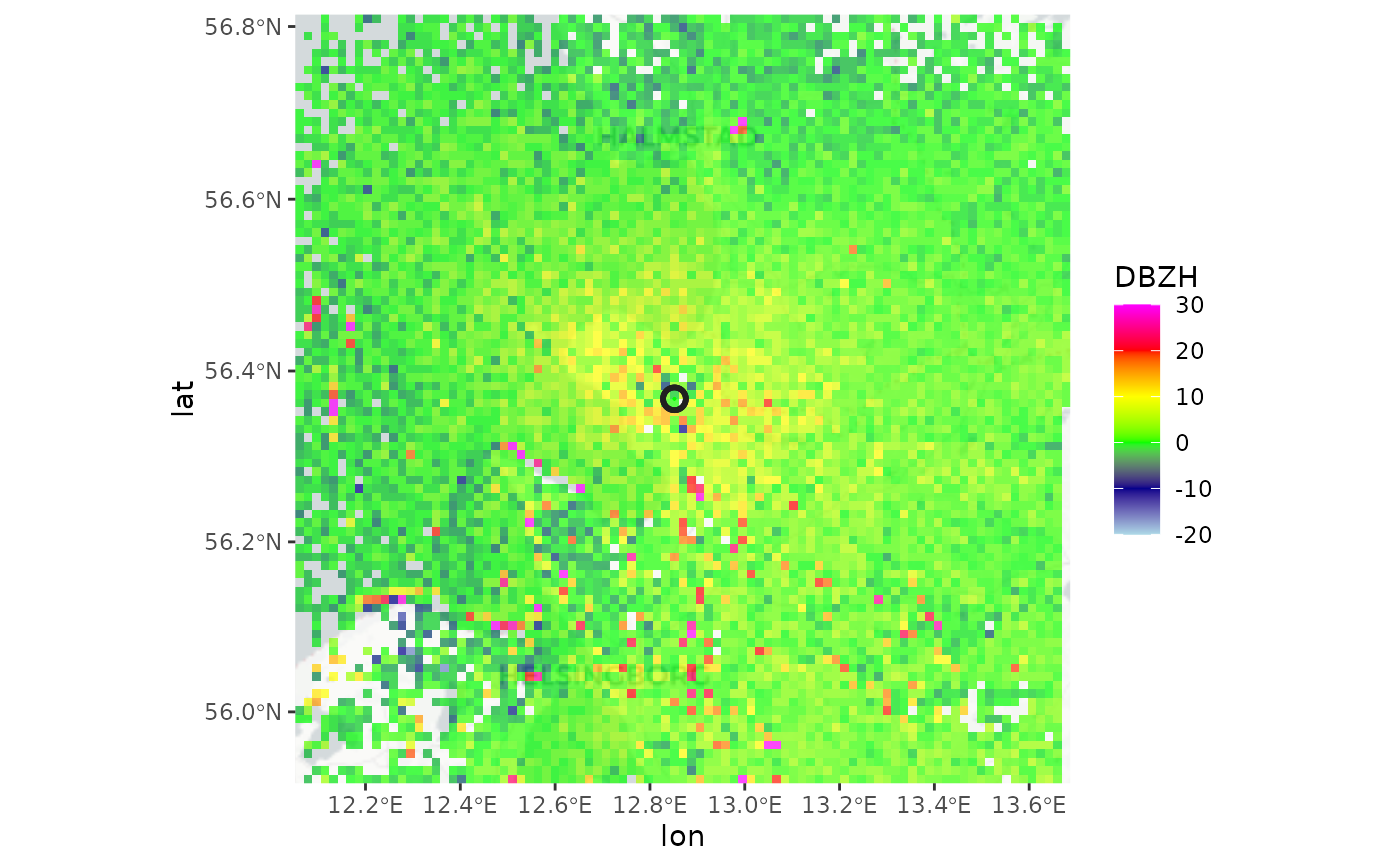Combines multiple plan position indicators (ppi) into a single ppi. Can be used to make a composite of ppi's from multiple radars.

composite_ppi(
x,
param = "all",
nx = 100,
ny = 100,
xlim,
ylim,
res,
crs,
raster = NA,
method = "max",
idp = 2,
idw_max_distance = NA,
coverage = FALSE
)

## Arguments

x

A list of ppi objects.

param

The scan parameter name(s) to composite. An atomic vector of character strings can be provided to composite multiple scan parameters at once. To composite all available scan parameters use 'all' (default).

nx

number of raster pixels in the x (longitude) dimension

ny

number of raster pixels in the y (latitude) dimension

xlim

x (longitude) range

ylim

y (latitude) range

res

numeric vector of length 1 or 2 to set the resolution of the raster (see res). If this argument is used, arguments nx and ny are ignored. Unit is identical to xlim and ylim.

crs

character or object of class CRS. PROJ.4 type description of a Coordinate Reference System (map projection). When 'NA' (default), an azimuthal equidistant projection with origin at the radar location is used. To use a WSG84 (lat,lon) projection, use crs="+proj=longlat +datum=WGS84"

raster

(optional) RasterLayer with a CRS. When specified this raster topology is used for the output, and nx, ny, res arguments are ignored.

method

string. Compositing method, one of "mean", "min", "max" or "idw". Provide a list of methods names of length(param) to apply different methods to each of the parameters.

idp

numeric. inverse distance weighting power.

idw_max_distance

numeric. Maximum distance from the radar to consider in inverse distance weighting. Measurements beyond this distance will have a weighting factor of zero.

coverage

logical. When TRUE adds an additional "coverage" parameter to the ppi with the number of PPIs covering a single composite ppi pixel.

## Value

A ppi.

## Details

This function composites multiple ppi objects into a ppi object that combines all data.

Either multiple ppi's of different scan elevation of the same radar may be combined, or ppi's of different radars can be composited.

Argument method determines how values of different ppi's at the same geographic location are combined.

"mean"

Compute the average value

"max"

Compute the maximum value. If ppi's are of the same radar and the same polar volume, this computes a max product, showing the maximum detected signal at that geographic location.

"min"

Compute the minimum value

"idw"

This option is useful primarily when compositing ppi's of multiple radars. Performs an inverse distance weighting, where values are weighted according to 1/(distance from the radar)^idp

The coordinates system of the returned ppi is a WGS84 (lat, lon) datum, unless a different crs is provided. If only res is provided, but no crs is set, res is in meter units and the origin of the composite ppi is set to the mean (lat, lon) location.

This function is a prototype and under active development

## Examples

# locate example volume file:
pvolfile <- system.file("extdata", "volume.h5", package = "bioRad")

# calculate a ppi for each elevation scan
my_ppis <- lapply(example_pvol\$scans, project_as_ppi)
#> Warning: CRS object has comment, which is lost in output; in tests, see
#> https://cran.r-project.org/web/packages/sp/vignettes/CRS_warnings.html
#> Warning: CRS object has comment, which is lost in output; in tests, see
#> https://cran.r-project.org/web/packages/sp/vignettes/CRS_warnings.html
#> Warning: CRS object has comment, which is lost in output; in tests, see
#> https://cran.r-project.org/web/packages/sp/vignettes/CRS_warnings.html

# overlay the ppi's, calculating the maximum value observed
# across the available scans at each geographic location
my_composite <- composite_ppi(my_ppis, method="max")

# \dontrun{
#> Map tiles by Stamen Design, under CC BY 3.0. Data by OpenStreetMap, under ODbL.

# plot the calculated max product on the basemap
map(my_composite, bm)
#> Warning: 'length(x) = 3 > 1' in coercion to 'logical(1)'
#> Warning: 'length(x) = 3 > 1' in coercion to 'logical(1)'
#> Warning: Discarded ellps WGS 84 in Proj4 definition: +proj=merc +a=6378137 +b=6378137 +lat_ts=0 +lon_0=0 +x_0=0 +y_0=0 +k=1 +units=m +nadgrids=@null +wktext +no_defs
#> Warning: Discarded datum WGS_1984 in Proj4 definition
#> Warning: CRS object has comment, which is lost in output; in tests, see
#> https://cran.r-project.org/web/packages/sp/vignettes/CRS_warnings.html
#> Warning: Discarded ellps WGS 84 in Proj4 definition: +proj=merc +a=6378137 +b=6378137 +lat_ts=0 +lon_0=0 +x_0=0 +y_0=0 +k=1 +units=m +nadgrids=@null +wktext +no_defs +type=crs
#> Warning: Discarded datum World Geodetic System 1984 in Proj4 definition# }